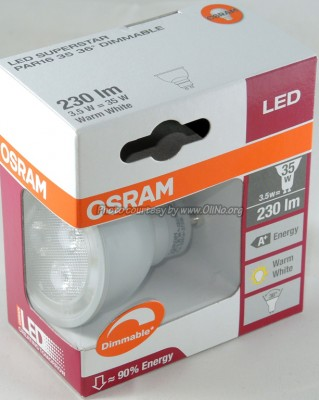# OliNo

Renewable Energy

## Osram – LED SUPERSTAR PAR16 36D 3.6-35W/827 GU10 dimmable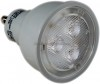Osram presents an LED spot light. The measurements from OliNo show that the lamp emits a warm white light with a color temperature of 2771 K. The lamp consumes 3.4 W and has a luminous flux of 233 lm. This results in an efficacy of 68 lm/W. The lamps energy category label is A+.

Also is tested on dim-ability with a (number of) 230 V dimmer(s).

See this overview for a comparison with other light bulbs.

### Summary measurement data dated 2016-06-04

parameter meas. result remark
Color temperature 2771 K warm white
Luminous intensity Iv 550.5 Cd Measured straight underneath the lamp.
Illuminance modulation index 0 % Measured with a light sensor looking at the lamp (angle not defined). Is a measure for the amount of flickering.
Beam angle 33 deg 33 deg is the beam angle for all C-planes since the lamp is symmetrical along its 1st axis.
Power P 3.4 W The net power consumed.
Power Factor 0.84 An electrical load with this power factor means that for every 1 kWh net energy consumed, there has been 0.65 kVAhr for reactive energy.
THD 28 % Total Harmonic Distortion.
Luminous flux 233 lm Measured with photogoniometer, calculation done as described in LM79-08.
Luminous efficacy 68 lm/W
EU2013-label classification A+ The energy class, from A++ (more efficient) to E (least efficient). This label is an update of the previous version, and compulsory from Sept 2013.
CRI_Ra 81 Color Rendering Index.
Qa_CQS 82.9 QCS (v9.0.3) is an improved indicator (over CRI) of how well colors are rendered.
Qg_CQS 0.93 Gamut Area Ratio.
Coordinates chromaticity diagram x=0.4084 en y=0.4537
Fitting GU10 This lamp is connected directly to the grid voltage.
PAR-value 5.1 uMol/s/m2 The number of photons seen by an average plant when it is lit by the light of this light bulb. Value valid at 1 m distance from light bulb.
PAR-photon efficacy 0.6 uMol/s/We The total emitted number of photons by this light, divided by its consumption in W. It indicates a kind of efficacy in generating photons.
Photon current 3.6 uMol/s The total number of photons in the light of this lamp.
S/P ratio 1.2 This factor indicates the amount of times more efficient the light of this light bulb is perceived under scotopic circumstances (low environmental light level).
D x H external dimensions 50 mm x 52 mm External dimensions of the lamp.
D luminous area 40 mm Dimensions of the luminous area (used in Eulumdat file). It is the surface of the front side.
General remarks The ambient temperature during the whole set of illuminance measurements was 25.3 – 25.6 deg C.Warm up effect: During the warmup time the illuminance varies during 15 minutes and decreases with 6 %.
During the warmup time the power doesn’t vary significantly (< 5 %).
The variation in efficacy (calculated as indication by simplydividing the illuminance by the power) during the warming up is -5 %. A very high negative value indicates a significant decrease for instance due to heating up of the lamp (decrease of lifetime).Voltage dependency: There is no (significant) dependency of the illuminance when the power voltage varies between 200 – 250 V AC.
There is no (significant) dependency of the consumed power when the power voltage varies between 200 – 250 V AC.

Also the dim-ability of this lamp has been tested, and the result is that the lamp is dim-able, see also the separate chapter in this test report.

At the end of the article an additional photo.

Eff-variation -5 % This is the variation in efficacy (calculated as indication by simply dividing the illuminance by the power) during the warming up. A very high negative value indicates a significant decrease for instance due to heating up of the lamp (decrease of lifetime).
Dimmable yes Info from manufacturer.

Melanopic effect factor
0.377 According to norm DIN SPEC 5031-100:2015-08.
Blue Light Hazard risk group 1 0=exempt, 1=low, 2 = moderate, 3=high risk.
form factor spot
EAN code 4008321882653
Brand Osram

### Overview table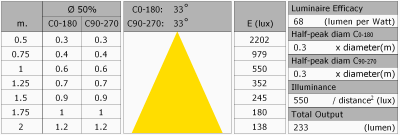Please note that this overview table makes use of calculations, use this data with care as explained on the OliNo site.

### EU 2013 Energy label classification

Since Sept 2013 these labels will be needed.

Important for the energy classification are the corrected rated power and the useful luminous flux.
The measured rated power is 3.4 W and might need to be corrected. The correction is dependent from the lamp type and whether or not the lamp control gear is included or not. The choice for this lamp is the following classification: Lamps with own control gear (external or internal). As a result the corrected rated power becomes: 3.4 W.
The luminous flux measured is 233 lm. The classification of this lamp needed to determine the useful flux is: Other directional lamps. Then the useful flux becomes 218 lm. Now a reference power can be calculated.

The energy efficiency coefficient is P_corr / P_ref = 0.15.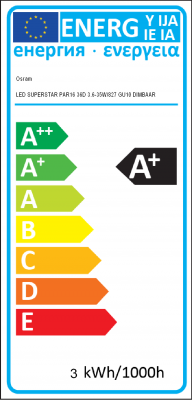EU energy label for this lampZip file with 6 EU energy labels of this lamp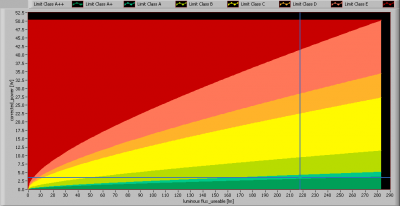The lamp’s performance in the lumen-Watt field, with the energy efficacy fields indicated.

### Eulumdat light diagram

This light diagram below comes from the program Qlumedit, that extracts these diagrams from an Eulumdat file.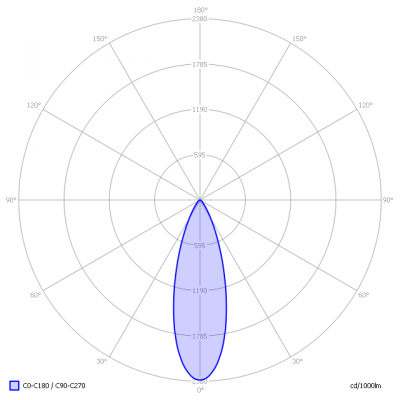The light diagram giving the radiation pattern.

The light diagram indicates the beam in the C0-C180 plane and in the plane perpendicular to that, the C90-C270 plane. These beams are equal as the lamp has symmetry over its first axis (the vertical axis).

### Illuminance Ev at 1 m distance, or luminous intensity Iv

Herewith the plot of the averaged luminous intensity Iv as a function of the inclination angle with the light bulb.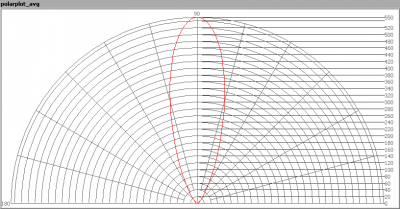The radiation pattern of the light bulb.

This radiation pattern is the average of the light output of the light diagram given earlier. Also, in this graph the luminous intensity is given in Cd.

These averaged values are used (later) to compute the lumen output.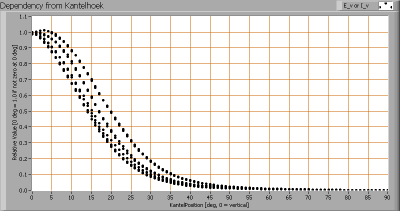Intensity data of every measured turn angle at each inclination angle.

This plot shows per inclination angle the intensity measurement results for each turn angle at that inclination angle. There normally are differences in illuminance values for different turn angles. However for further calculations the averaged values will be used.

When using the average values per inclination angle, the beam angle can be computed, being 33 deg for the C0-C180 plane and 33 deg for the C90-C270 plane.

### Luminous flux

With the averaged illuminance data at 1 m distance, taken from the graph showing the averaged radiation pattern, it is possible to compute the luminous flux.

The result of this computation for this light spot is a luminous flux of 233 lm.

### Luminous efficacy

The luminous flux being 233 lm, and the consumed power of the lamp being 3.4 Watt, results in a luminous efficacy of 68 lm/Watt.

### Electrical properties

The power factor is 0.84. An electrical load with this power factor means that for every 1 kWh net energy consumed, there has been 0.65 kVAhr for reactive energy.

 Lamp voltage 230.15 V Lamp current 0.018 A Power P 3.4 W Apparent power S 4.1 VA Power factor 0.84

Of this lamp the voltage across and the resulting current through it are measured and graphed.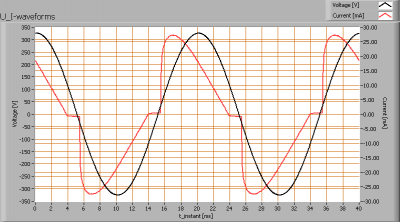Voltage across and current through the lightbulb

This current waveform has been checked on requirements posed by the norm IEC 61000-3-2:2006 (including up to A2:2009).Harmonics in the current waveform and checked against IEC61000-3-2:2006 and A2:2009

When the consumed power is <= 25 W there are no limits for the harmonics.

The Total Harmonic Distortion of the current is computed and its value is 28 %.

### Color temperature and Spectral power distribution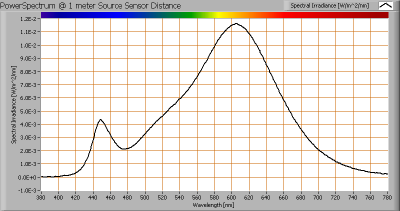The spectral power distribution of this light bulb, energies on y-axis valid at 1 m distance.

The measured color temperature is 2771 K which is warm white.

This color temperature is measured straight underneath the light bulb. Below a graph showing the color temperature for different inclination angles.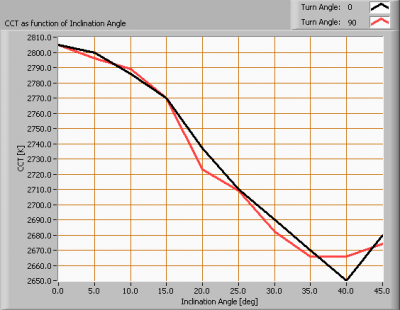Color temperature as a function of inclination angle.

The color temperature is given for inclination angles up to 45 deg. Beyond that value the illuminance is so low (< 0.10 lux) that it has not been used for color determination of the light.

For the C0-C180 plane: the beam angle of 33 deg is equivalent to 16.5 deg inclination angle,which is the area where most of the light falls within. The maximum variation of color temperature in this inclination area is about 2 %.

For the C90-C270 plane: the beam angle of 33 deg is equivalent to 16.5 deg inclination angle,which is the area where most of the light falls within. The maximum variation of color temperature in this inclination area is about 2 %.

### PAR value and PAR spectrum

To make a statement how well the light of this light bulb is for growing plants, the PAR-area needs to be determined.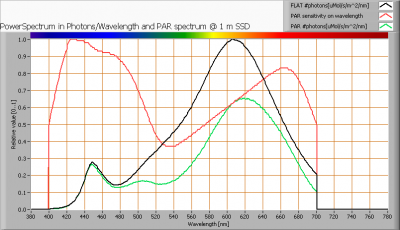The photon spectrum, then the sensitivity curve and as result the final PAR spectrum of the light of this light bulb

parameter value unit
PAR number 5.1 uMol/s/m^2
PAR photon current 2.1 uMol/s
PAR photon efficacy 0.6 uMol/s/W

The PAR efficiency is 64 % (valid for the PAR wave length range of 400 – 700 nm). This is the maximum percentage of the total of photons in the light that is effectively used by the average plant (since the plant might not take 100 % of the photons at the frequency where its relative sensitivity is 100 %).

### S/P ratio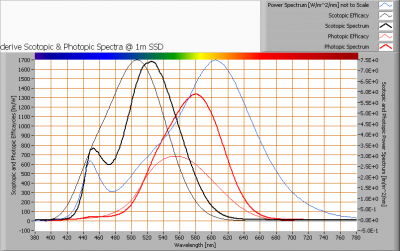The power spectrum, sensitivity curves and resulting scotopic and photopic spectra (spectra energy content defined at 1 m distance).

The S/P ratio of the light coming from this lamp is 1.2.

### Chromaticity diagram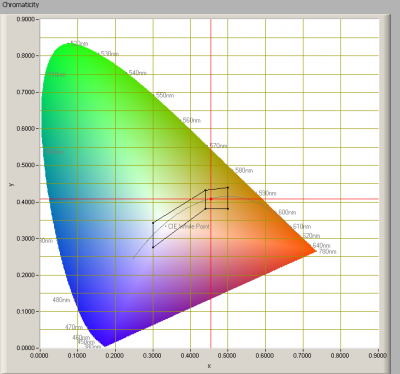The chromaticity space and the position of the lamp’s color coordinates in it.

The point of the light in this diagram is inside the area indicated with class B. This area indicates an area for signal lamps.

The color coordinates are x=0.4084 and y=0.4537.

### Color Rendering Index (CRI) or also Ra

Herewith the image showing the CRI as well as how well different colors are represented (rendered). The higher the number, the better the resemblance with the color when a black body radiator would have been used (the sun, or an incandescent lamp)

Each color has an index Rx, and the first 8 indexes (R1 .. R8) are averaged to compute the Ra which is equivalent to the CRI.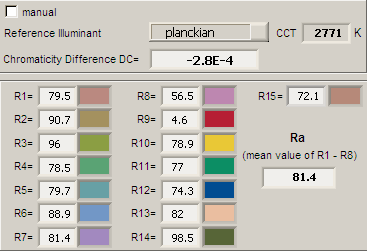CRI of the light of this lightbulb.

This value of 81 indicates how well the light of this lamp can render well a set of reference colors, this in comparison with the light of a reference source (for color temperatures < 5000K a black radiator is used as reference and for color temperatures > 5000K the sun or the light outside during the day).

The value of 81 is bigger than the value of 80 that is considered as a minimum for working areas in general.

Note: the chromaticity difference is -0.0003 and indicates the distance to the Planckian Locus. There is a value mentioned of max 5.4E-3 in section 5.3 of CIE 13.3-1995 however not further explanation of it.
An other reference with signal lights as a reference is given in the chromaticity diagram.

### Color Quality Scale

QCS (v9.0.3) is an improved indicator (over CRI) of how well colors are rendered.CQS-values of the light of this light bulb.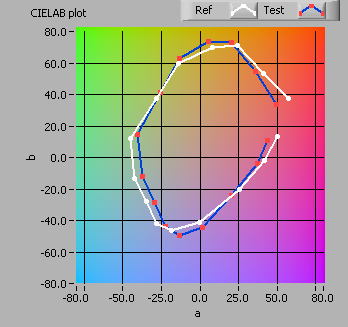CQS-values for the light of this light bulb compared with those of a reference source with the same color temperature.

### Voltage dependency

The dependency of a number of lamp parameters on the lamp voltage is determined. For this, the lamp voltage has been varied and its effect on the following light bulb parameters measured: illuminance E_v [lx], the lamppower P [W] and the luminous efficacy [lm/W] (this latter is estimated here by dividing the found E_v value by P).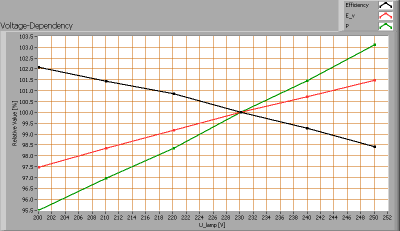Lamp voltage dependencies of certain light bulb parameters

There is no (significant) dependency of the illuminance when the power voltage varies between 200 – 250 V AC.
There is no (significant) dependency of the consumed power when the power voltage varies between 200 – 250 V AC.

When the voltage varies abruptly with + or – 5 V AC then this results in a variation of the illuminance of maximally 0.4 %. This difference in illuminance is not visible (when it occurs abruptly).

### Warm up effects

After switch on of a cold lamp, the effect of heating up of the lamp is measured on illuminance E_v [lx], the lamppower P [W] and the luminous efficacy [lm/W].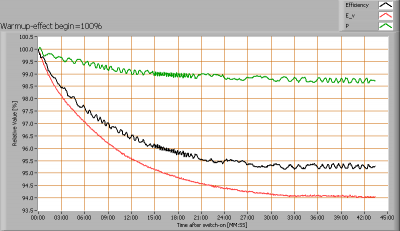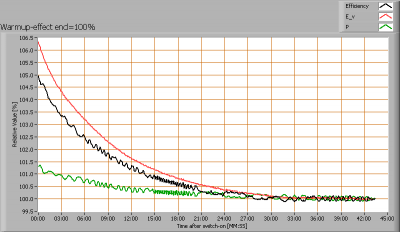Effect of warming up on different light bulb parameters. In the first graph the 100 % level is put at begin, and in the last graph the 100 % level is put at the end.

During the warmup time the illuminance varies during 15 minutes and decreases with 6 %.
During the warmup time the power doesn’t vary significantly (< 5 %).
The variation in efficacy (calculated as indication by simplydividing the illuminance by the power) during the warming up is -5 %. A very high negative value indicates a significant decrease for instance due to heating up of the lamp (decrease of lifetime).

### Measure of flickering

An analysis is done on the measure of flickering of the light output by this light bulb.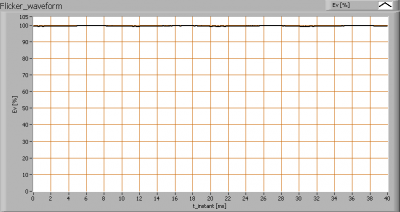The measure of fast illuminance variation of the light of the light bulb

parameter value unit
Flicker frequency 100.2 Hz
Illuminance modulation index 0 %
Flicker index 0.001 [-]

The illuminance modulation index is computed as: (max_Ev – min_Ev) / (max_Ev + min_Ev).

### Dim-ability

The lamp has been tested on the following dimmer(s): the Busch Jaeger 6523 U dimmer.

The Busch Jaeger 6523 U.Dimming with the Busch Jaeger 6523 U.

When inserting the dimmer at its no dimming position and the comparing with the situation without dimmer, then the influence on the following parameters is measured (negative value is increase):
– the illuminance: 8 %;
– the consumed power: -0 %.

The intensity is variable in the mechanical area between 0.0 – 100.0 %.
The dimmer set in that area results in a variation of the illuminance between 0 – 100 % (note that in that 100 % the drop in illuminance when inserting the dimmer is not counted, see for that value above).

There is almost no effect on the color temperature when dimming is increased.

The remaining power consumption at maximal dimming position is 0.5 W.

### Melanopic effect

The melanopic effect shows the level of impact the light of this lamp can have on the day-night rhythm of human beings (as well as the suppression of melatonin production).
The important parameters (according to norm DIN SPEC 5031-100:2015-08):

 melanopic effect factor 0.377 kmel trans (25 years) 1.043 kmel trans (32 years) 1 kmel trans (50 years) 0.862 kmel trans(75 years) 0.645 kmel trans(90 years) 0.523 kpupil(25 years) 1.088 kpupil(32 years) 1 kpupil(50 years) 0.792 kpupil(75 years) 0.543 kpupil(90 years) 0.416

### Blue Light Hazard

The amount of blue light and the harm it can cause on the retina has been determined. Herewith the results.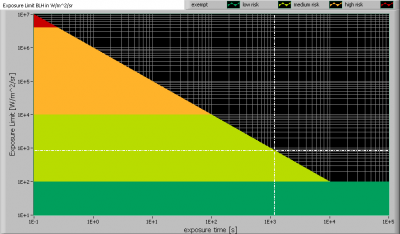The level of blue light of this lamp related to the exposure limit and the different classification areas.

 L_lum0 [mm] 15 Dimension of brightest part of lamp in C0-C180 direction. L_lum90 [mm] 15 Dimension of brightest part of lamp in C90-C270 direction. SSD_500lx [mm] 1049 Calculated distance where Ev = 500 lux. This computation is valid when it is in the far field of the lamp. Note: if this value 200 mm then the distance of 200 mm is taken as proposed in the norm IEC 62471:2006. Start of far field [mm] 106 Minimum distance at which the lamp can be seen as apoint source. In this area the Ev is linearly dependent from (1/distance)2. 300-350 nm values stuffed with 0s yes In the event OliNo has measured with a SpB1211 spectrometer without UV option then the irradiance data of 300-349 nm is missing. For lamps where there is already no energy content near 350 nm, the values 300-349 can also be set at zero then. alphaC0-C180 [rad] 0.014 (Apparent) source angle in C0-C180 direction. alphaC90-C270 [rad] 0.014 (Apparent) source angle in C90-C270 direction. alphaAVG [rad] 0.014 Average (apparent) source angle. If average >= 0.011 rad then the exposure limit is computed with radiance Lb. Otherwise with irradiance Eb. Exposure value [W/m^2/sr] 8.50E+2 Blue Light Hazard value for this lamp, measured straight underneath the lamp. Computation is referenced to Lb. Blue Light Hazard risk group 1 0=exempt, 1=low, 2 = moderate, 3=high risk.

### Extra